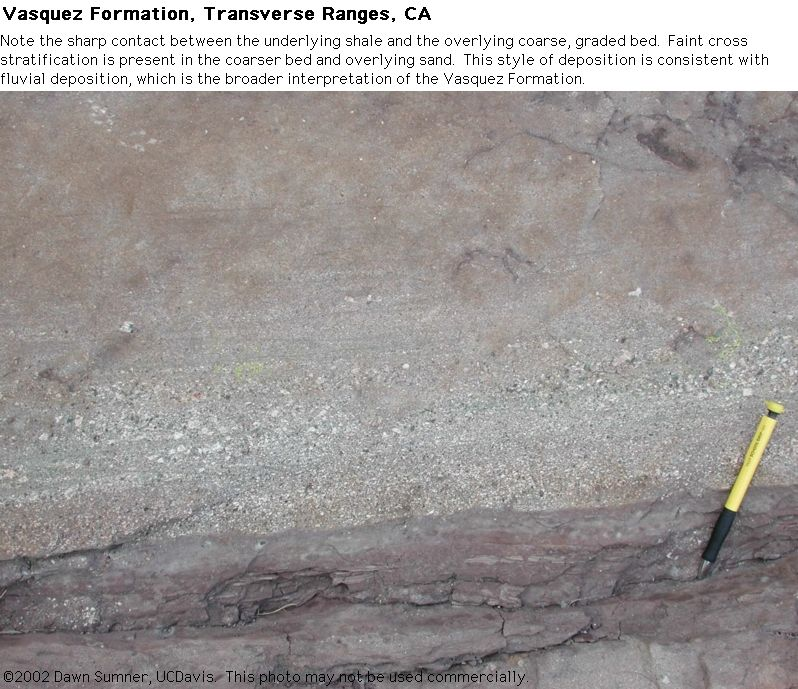# 6.2.7: Subaqueous Bedforms and Cross Stratification

$$\newcommand{\vecs}{\overset { \rightharpoonup} {\mathbf{#1}} }$$ $$\newcommand{\vecd}{\overset{-\!-\!\rightharpoonup}{\vphantom{a}\smash {#1}}}$$$$\newcommand{\id}{\mathrm{id}}$$ $$\newcommand{\Span}{\mathrm{span}}$$ $$\newcommand{\kernel}{\mathrm{null}\,}$$ $$\newcommand{\range}{\mathrm{range}\,}$$ $$\newcommand{\RealPart}{\mathrm{Re}}$$ $$\newcommand{\ImaginaryPart}{\mathrm{Im}}$$ $$\newcommand{\Argument}{\mathrm{Arg}}$$ $$\newcommand{\norm}{\| #1 \|}$$ $$\newcommand{\inner}{\langle #1, #2 \rangle}$$ $$\newcommand{\Span}{\mathrm{span}}$$ $$\newcommand{\id}{\mathrm{id}}$$ $$\newcommand{\Span}{\mathrm{span}}$$ $$\newcommand{\kernel}{\mathrm{null}\,}$$ $$\newcommand{\range}{\mathrm{range}\,}$$ $$\newcommand{\RealPart}{\mathrm{Re}}$$ $$\newcommand{\ImaginaryPart}{\mathrm{Im}}$$ $$\newcommand{\Argument}{\mathrm{Arg}}$$ $$\newcommand{\norm}{\| #1 \|}$$ $$\newcommand{\inner}{\langle #1, #2 \rangle}$$ $$\newcommand{\Span}{\mathrm{span}}$$$$\newcommand{\AA}{\unicode[.8,0]{x212B}}$$

I have divided these by type of bedform and cross stratification. The examples are both fluvial and marine.

# Planar Cross StratificationFigure $$\PageIndex{1}$$: Ancient Planar Cross StratificationFigure $$\PageIndex{2}$$: Ancient Planar Cross StratificationFigure $$\PageIndex{3}$$: Single set of cross strata in a planar bed

# Trough Cross StratificationFigure $$\PageIndex{4}$$: Ancient Trough Cross StratificationFigure $$\PageIndex{5}$$: Ancient Trough Cross StratificationFigure $$\PageIndex{6}$$: Ancient Trough Cross StratificationFigure $$\PageIndex{7}$$: Plan or Map View of Ancient Trough Cross Stratification

# Herringbone Cross StratificationFigure $$\PageIndex{8}$$: Herringbone cross stratification

# Hummocky Cross StratificationFigure $$\PageIndex{9}$$: Hummocky cross stratificationFigure $$\PageIndex{10}$$: Hummocky cross stratification

# Cross Stratification from Bar MigrationFigure $$\PageIndex{11}$$: Recent Alluvial Deposits (Terrace at Redrock State Park, CA)Figure $$\PageIndex{12}$$: Recent Alluvial Deposits (Terrace at Redrock State Park, CA)Figure $$\PageIndex{13}$$: Recent Fluvial Deposits (Alsek River, Alaska)Figure $$\PageIndex{14}$$: Stratification Due to Bar MigrationFigure $$\PageIndex{15}$$: Same outcrop as above, but also showing channel fill deposits above the sandstones deposited by the bar.Figure $$\PageIndex{16}$$: Quarry wall showing fluvial cross stratification, probably due to channel migration and bar accretion.Figure $$\PageIndex{17}$$: Extension of the same quarry wall as above

# Dune Cross Stratification

(that does not fit nicely into a specific category)Figure $$\PageIndex{18}$$: Climbing DunesFigure $$\PageIndex{19}$$: Ancient Dune Cross StratificationFigure $$\PageIndex{20}$$: Cross Stratified GravelFigure $$\PageIndex{21}$$: Graded bedding with faint cross stratification deposited in a fluvial channelFigure $$\PageIndex{22}$$: Cross stratification in a channel fill in a subaqueous turbidite fanFigure $$\PageIndex{23}$$: Cross stratification in a channel fill in a subaqueous turbidite fan

The following photographs are of large-scale cross stratification that was deposited by the migration of giant dunes and/or large fluvial bars. Detailed analysis of the regional characteristics of these deposits would be required to distinguish between dune and bar deposition in many cases, and both might be present.Figure $$\PageIndex{24}$$: Large Cross StratificationFigure $$\PageIndex{25}$$: Large Cross StratificationFigure $$\PageIndex{26}$$: Cross stratified sediment deposited by a migrating bar based on the abundance of more planar laminae that would be characteristic of a channel bottom.Figure $$\PageIndex{27}$$: Different view of the bedform in the previous photo

The following photographs were taken by Jeff Mount during his Roy Shlemon Course in Applied Watershed Science class trip down the Copper River, Alaska and Yukon (see the Shlemon class web page). The river shown below was dammed by ice from the toe of a glacier, which created a very large lake. The ice dam broke catastrophically, producing a huge flood. The flood waters transported very large volumes of sediment in huge dunes. After normal river processes resumed, the river eroded into the flood deposits, exposing the cross stratification in these impressive dunes.Figure $$\PageIndex{28}$$: Lake shoreline terraces from the lakeFigure $$\PageIndex{29}$$: Lake shoreline terraces from the lake and cross stratification in the river bank. This cross stratification may have been deposited by the flood waters.​​​​​​​Figure $$\PageIndex{30}$$: Remnants of giant dunes that migrated during the floodFigure $$\PageIndex{31}$$: Large dune cross stratification and remnants of giant dunes​​​​​​​Figure $$\PageIndex{32}$$: Large dune cross stratification and remnants of giant dunes​​​​​​​